# Precalculus : Graph a Quadratic Function Using Intercepts, Vertex, and Axis of Symmetry

## Example Questions

### Example Question #1 : Graphing Quadratic Functions

Find the vertex, roots, and thevalue that the line of symmetry falls on of the function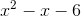.

vertex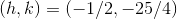, the roots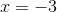and, and the axis of symmetry would fall on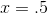.

vertex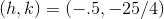, the rootsand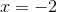, and the axis of symmetry would fall on x=.5.

vertex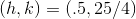, the rootsand, and the axis of symmetry would fall on.

vertex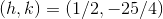, the rootsand, and the axis of symmetry would fall on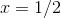.

vertex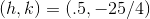, the rootsand, and the axis of symmetry would fall on.

vertex, the rootsand, and the axis of symmetry would fall on.

Explanation:

All quadratic functions have a vertex and many cross the x axis at points called zeros or roots. If we know the vertex and its zeros, quadratic functions become very easy to draw since the vertex is also a line of symmetry (the zeros are equidistant from the vertex on either side).

Factor the equationto get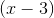and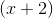. Thus, the roots are 3 and -2.

The vertex can be found by using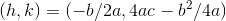.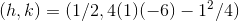simplify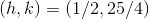.

The axis of symmetry is halfway between the two roots, or simply the x coordinate of the vertex. So the axis of symmetry lies on x=1/2. To graph, draw a point at the coordinate pair of the vertex. Then draw points on the x axis at the roots, and finally, trace upwards from the vertex through the roots with a gentle curve.

### Example Question #2 : Graphing Quadratic Functions

Which of the following functions matches the provided parabolic graph?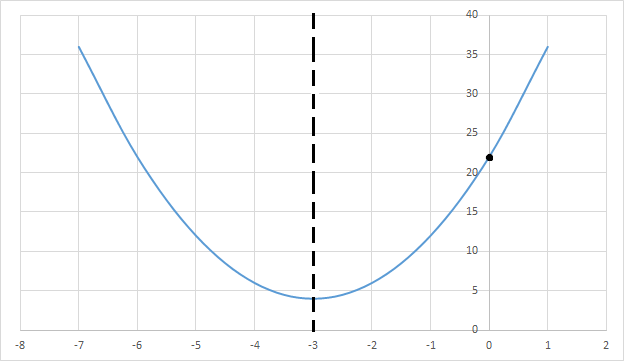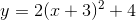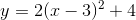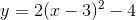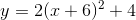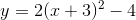Explanation:Finding the vertex, intercept and axis of symmetry are crucial to finding the function that corresponds to the graph:

The vertex form of a quadratic function is written as: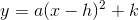and the coordinates for the vertex are: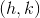Looking at the graph and the position of the axis of symmetry, the vertex is positioned at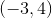, leaving us with an equation so far of: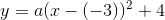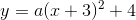While we don't know a right away,is the only option that really works. The y-intercept is at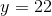and we can plug that into the formula to confirm that this is the correct function: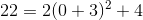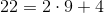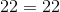### Example Question #3 : Graphing Quadratic Functions

Which of the following is an equation for the parabola represented in the graph below?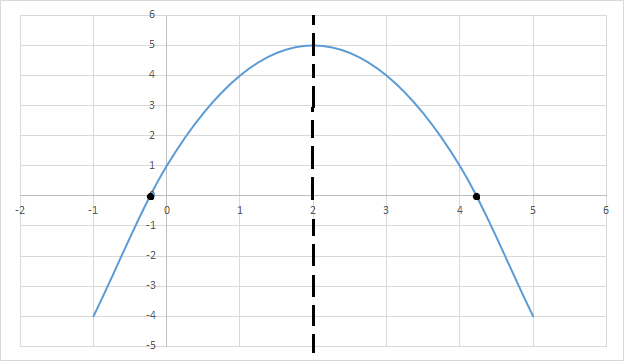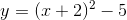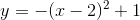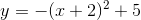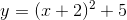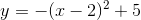Explanation:

Immediately we can tell that the equation has a negative coefficient, because the parabola opens downward, forming an umbrella shape. Based upon the information given in the figure, we can use the intercepts, axis of symmetry, and the vertex to identify the equation of the parabola. Let's observe the vertex form of a parabola written as the following:In this equation,is the vertex of the parabola, anddetermines whether the parabola opens upwards or downwards. The axis of symmetry is atand the vertex is located at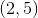, which we can plug into the following function: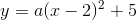We know thatis negative because of the position of the parabola.### All Precalculus Resources